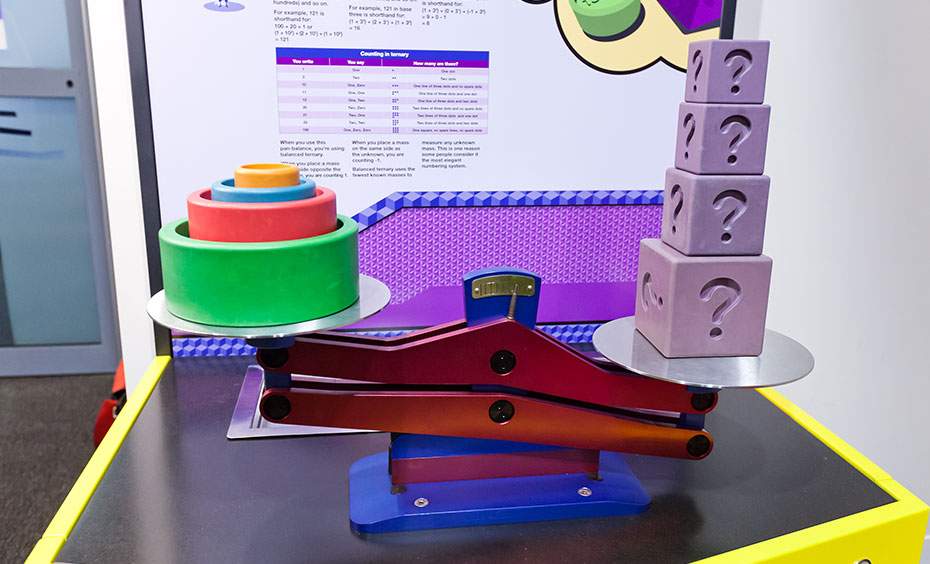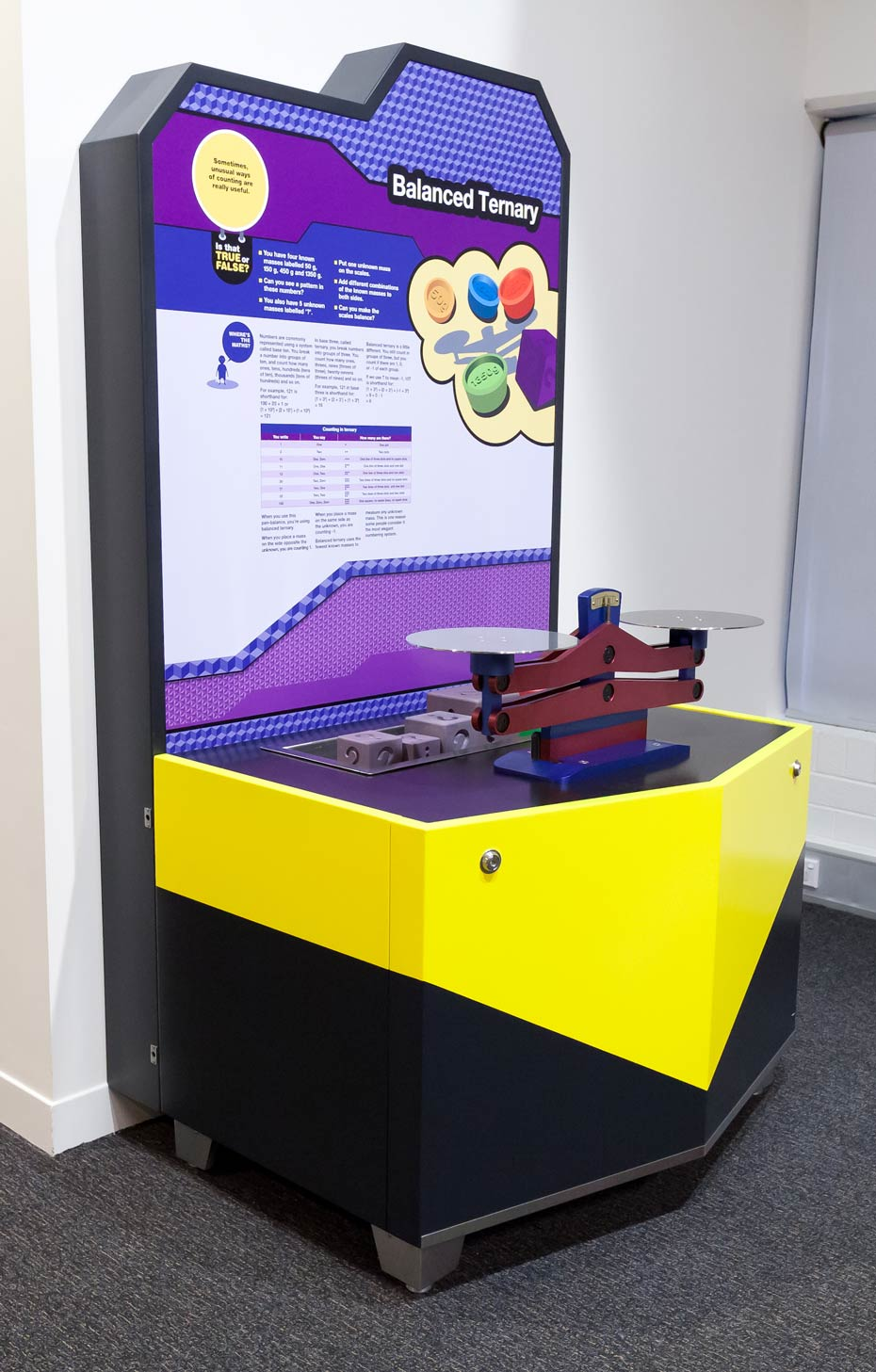# Balanced TernaryThe ternary counting system is based on 'threes', while our traditional decimal counting system is based on 'tens'.

## How it works

Work out the mass of an unknown object by comparing it to a series of known masses ( 50 g, 150 g, 450 g and 1350 g) using a novel counting system known as balanced ternary.

## Things to try or ask around the exhibit

• What numerical pattern can you pick up in the known masses 50 g, 150 g, 450 g and 1350 g?
• What's the smallest number of masses you can use to make the unknown object balance?
• If you count to 100, what base number is that counting system based on?

## Background

This exhibit combines problem solving with a model of the ternary counting system.

Numbers are commonly represented using a system called base ten. You break a number into groups of ten, and count how many ones, tens, hundreds (tens of ten), thousands (tens of hundreds) and so on. For example, 121 is shorthand for 100 + 20 + 1 or (1×102) + (2×101) + (1×100).

In base three, called ternary, you break numbers into groups of three. You count how many ones, threes, nines (threes of three), twenty-sevens (threes of nines). For example, 121 in base three is shorthand for (1×32) + (2×31) + (1×30) = 16.